## Selina Concise Mathematics Class 10 ICSE Solutions Chapter 20 Cylinder, Cone and Sphere (Surface Area and Volume) Ex 20A

These Solutions are part of Selina Concise Mathematics Class 10 ICSE Solutions. Here we have given Selina Concise Mathematics Class 10 ICSE Solutions Chapter 21 Trigonometrical Identities Ex 21A

Other Exercises

Question 1.

The height of a circular cylinder is 20 cm and the radius of its base is 7 cm. Find :
(i) the volume
(ii) the total surface area.
Solution:
Height of cylinder (h) =  20cm
and radius of its base (r) = 7 cm
(î) Volurne=πr²h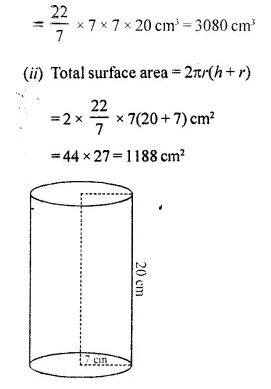Question 2.
The inner radius of a pipe is 2.1 cm. How much water can 12 m of this pipe hold ?
Solution:
Inner radius of a pipe (r) = 2.1 cm
and length of pipe (h) = 12 m = 1200 cm
∴ Volume of water in it = πr2h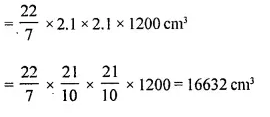Question 3.
A cylinder of circumference 8 cm and length 21 cm roils without sliding for 4 $$\frac { 1 }{ 2 }$$ seconds at the rate of 9 complete rounds per second. Find:
(i) the distance travelled by the cylinder in 4 $$\frac { 1 }{ 2 }$$ seconds, and
(ii) the area covered by the cylinder in 4 $$\frac { 1 }{ 2 }$$ seconds.
Solution:
Circumference of a cylinder = 8 cm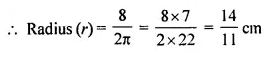Length of cylinder (h) = 21 cm
It takes 9 complete rounds per second
∴ Curved surface area = 2πrh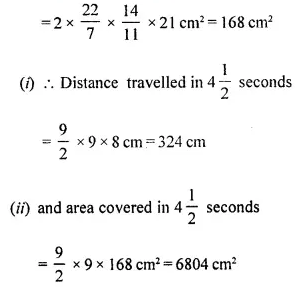Question 4.
How many cubic metres of earth must be dug out to make a well 28 m deep and 2.8 m in diameter ? Also, find the cost of plastering its inner surface at ₹4.50 per sq. metre.
Solution:Question 5.
What length of solid cylinder 2 cm in diameter must be taken to recast into a hollow cylinder of external diameter 20 cm, 0.25 cm thick and 15 cm long ?
Solution:
Diameter of solid cylinder = 2 cm
∴ Radius (r) = $$\frac { 2 }{ 2 }$$ = 1 cm
Let length (h) = x cm
∴ Volume = πr²h = π x 1 x 1 x x
= πx cm3       …(i)
External diameter of hollow cylinder = 20cm
∴ External radius =  $$\frac { 20 }{ 2 }$$ = 10 cm
Thickness of cylinder = 0.25 cm
∴ Innerradius= 10-0.25 = 9.75 cm
Length = 15 cm
∴ Volume = π(R2 – r2) x h
= π(R + r)(R-r) x h
= π(10 + 9.75)(10-9.75) x 15 cm3
= πx 19.75 x 0.25 x 15 cm3    ………(ii)
Comparing (i) and (ii), we get
∴ π x 19.75 x 0.25 x 15 = π x
x = 19.75 x 0.25 x 15 cm
= 74.0625 = 74.06 cm

Question 6.
A cylinder has a diameter of 20 cm. The area of the curved surface is 100 cm2 (sq. cm). Find:
(i) the height of the cylinder correct to one decimal place.
(ii) the volume of the cylinder correct to one decimal place.
Solution: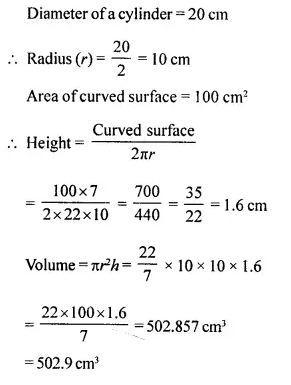Question 7.
A metal pipe has a bore (inner diameter) of 5 cm. The pipe is 5 mm thick all round. Find the weight, in kilogram, of 2 metres of the pipe if 1 cm3 of the metal weighs 7.7 g.
Solution: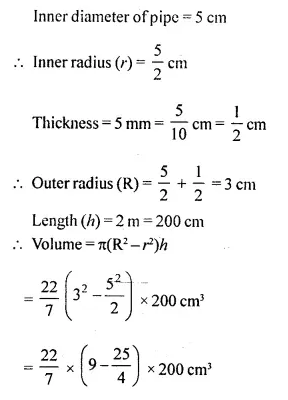Question 8.
A cylindrical container with diameter of base 42 cm contains sufficient water to submerge a rectangular solid of iron with dimensions 22 cm x 14 cm x 10.5 cm. Find the rise in the. level of the water when the solid is submerged.
Solution:
Diameter of base of a cylindrical container =42 cm
∴ Radius = $$\frac { 42 }{ 2 }$$ = 21 cm
Size of rectangular solid = 22cmx 14cmx 10.5 cm
∴ Volume of solid = 3234 cm3
∴ Height of water level raise in the container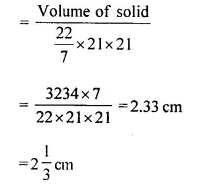Question 9.
A cylindrical container with infernal radius of its base 10 cm’, contains water up”to a height of 7 cm. Find the area of the wet surface of the cylinder.
Solution:
Internal radius of cylindrical container (r) = 10cm
Water upto height (h) = 7 cm
∴ Area of wet surface by the water of the container = 2πrh
= π x 19.75 x 0.25 x 15 cm3                       …(ii)
Comparing (i) and (ii), we get
∴ π x 19.75 x 0.25 x 15 = πx
x = 19.75×0.25x 15 cm
= 74.0625
= 74.06 cm

Question 10.
Find the total surface area of an open pipe of length 50 cm, external diameter 20 cm and internal diameter 6 cm.
Solution:
Length of open pipe (h) = 50 cm
External diameter=20 cm
and internal diameter = 6 cm
∴ External radius (R) = $$\frac { 20 }{ 2 }$$ = 10 cm
and internal radius (r) = $$\frac { 6 }{ 2 }$$ = 3 cm
∴ Total surface area of the open pipe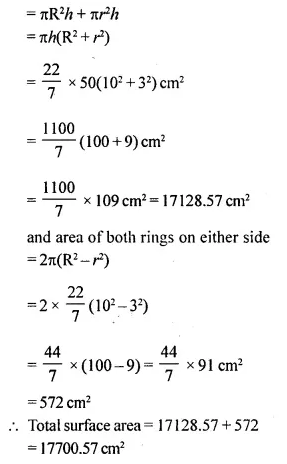Question 11.
The height and the radius of the base of a cylinder are in the ratio 3:1. If its volume is 1029 πcm3; find its total surface area.
Solution:
Ratio in height and radius of cylinder = 3:1
Let height = 3x
∴ Volume = πr2h = π x 3x x x2 = 3πx3
∴ 3πt3= 1029π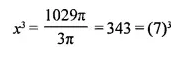∴ x = 7
∴ Height = 7 x 3=21 cm
Now total surface area = 2πr2 + 2 πrh
= 2 πr (r + h)
= 2 x $$\frac { 22 }{ 7 }$$ x 7(7 + 21)
=44 x 28 = 1232 cm2

Question 12.
The radius of a solid right circular cylinder increases by 20% and its height decreases by 20%. Find the percentage change in its volume.
Solution:
Let radius of the cylinder (r) = 100 cm
and height (h) = 100 cm
∴ Volume = πr2h =π( 100)2 x 100 cm3 = 1000000π cm3
Now radius (R) = 100 + 20 = 120 cm
and new height (h) = 100 – 20 = 80 cm
∴ Volume = π(120)2 x 80
= π x 14400 x 80cm3= 152000π cm3
Percentage change (increase) in the volume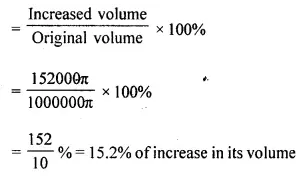Question 13.
The radius of a solid right circular cylinder decreases by 20% and its height increases by 10%. Find the percentage change in its:
(i) volume
(ii) curved surface area
Solution:
Let radius of the cylinder = 100 cm
and height = 100 cm
∴ Volume = πr2h
= πx 100 x 100 x 100 cm3
= 1000000πcm3
and Curved surface area = 2πrh
= 2 x π x 100 x 100 cm2
=20000π cm2
Decreased radius = 100 – 20 = 80 cm
and increased height = 100 + 10 = 110 cm
∴ Increased volume = π x 80 x 80 x 110 cm3 = 704000π cm3
and increased curved surface =2 x π x 80 x 110 cm2 = 17600πcm2
⇒  Decrease in volume = 1000000π – 704000π = 296000π cm3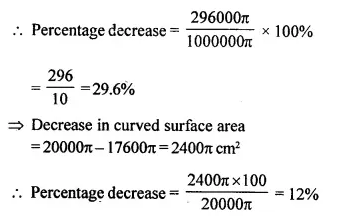Question 14.
Find the minimum length in cm and correct to nearest whole number of the thin metal sheet required to make a hollow and closed cylindrical box of diameter 20 cm and height 35 cm. Given that the width of the metal sheet is 1 m. Also, find the cost of the sheet at the rate of ₹56 per m.
Find the area of metal sheet required, if 10% of it is wasted in cutting, overlapping, etc.
Solution:
Diameter of the hollow closed cylinder =20 cm
∴ Radius (r) = $$\frac { 20 }{ 2 }$$
and height (h) = 35 cm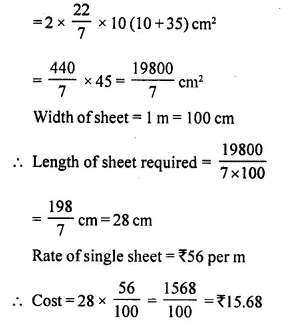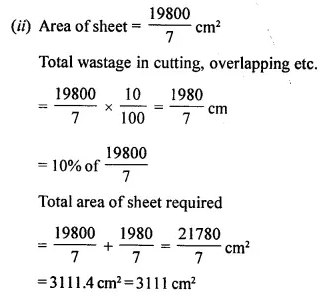Question 15.
3080 cm3 of water is required to fill a cylindrical vessel completely and 2310 cm3 of water is required to fill it upto 5 cm below the top. Find:
(ii) height of the vessel.
(iii) wetted surface area of the vessel when it is half-filled with water.
Solution:
Volume of water to fill a cylindrical vessel = 3080 cm3
Volume of water to fill it upto 5 cm below = 2310 cm2
Volume of water for 5 cm height =3080- 2310 = 770cm3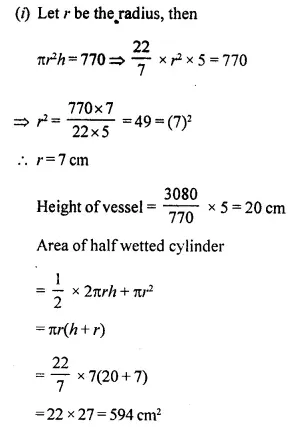Question 16.
Find the volume of the largest cylinder formed when a rectangular piece of paper 44 cm by 33 cm is rolled along it:
(i) shorter side.
(ii) longer side.
Solution:
Length of rectangular sheet = 44 cm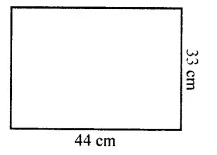(i) Folding along shorter side i.e., 33cm
∴ Circumference of cylinder = 33cmQuestion 17.
A metal cube of side 11 cm is completely submerged in water contained in a cylindrical vessel with diameter 28 cm. Find the rise in the level of water.
Solution:
Side of a cube = 11 cm
∴ Volume = (Side)3 = 11 x 11 x 11 cm3 = 1331 cm3
Diameter of cylinder = 28 cm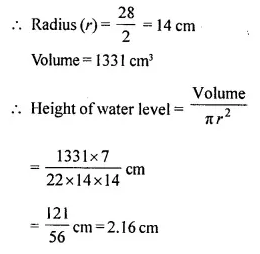Question 18.
A circular tank of diameter 2 m is dug and the earth removed is spread uniformly all around the tank to form an embankment 2 m in width and 1.6 m in height. Find the depth of the circular tank.
Solution:
Diameter of circular tank = 2m
Width of embankment = 2 m
Height = 1.6 m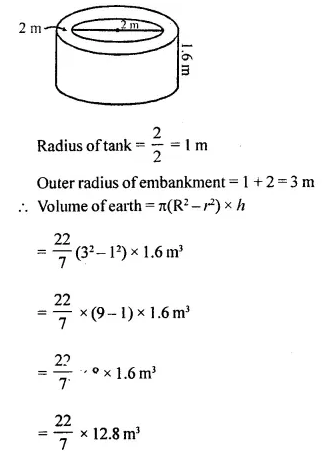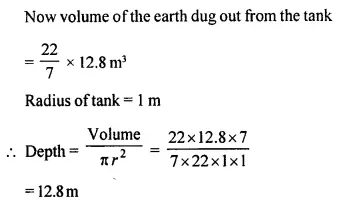Question 19.
The sum of the inner and the outer curved surfaces of a hollow metallic cylinder is 1056 cm2 and the volume of material in it is 1056 cin3. Find its internal and external radii. Given that the height of the cylinder is 21 cm.
Solution:
Sum of outer and inner surface area of a hollow cylinder = 1056 cm2
Volume of metal used =1056 cm3
Height of cylinder (h) = 21 cm
Height = 21 cm
We are given
Outer surface + Inner surface = 1056 cm2
Volume = πR2h – πr2h =1056 cm3
Now, 2πRh + 2πrh =1056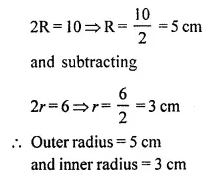Question 20.
The difference between the outer curved surface area and the inner curved surface area of a hollow cylinder is 352 cm2. If its height is 28 cm and the volume of material in it is 704 cm3; find its external curved surface area.
Solution:
Difference in outer and inner curved surface of a hollow cylinder = 352 cm2
Height (h) = 28 cm
Volume of material used = 704 cm3
∴ 2πRh-2πrh = 352
2πh(R-r) = 352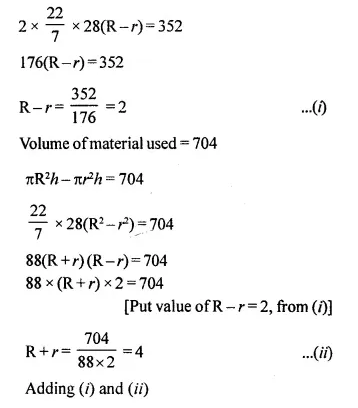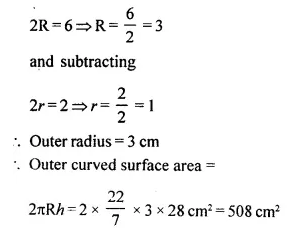Question 21.
The sum of the height and the radius of a solid cylinder is 35 cm and its total surface area is 3080 cm2; find the volume of the cylinder.
Solution: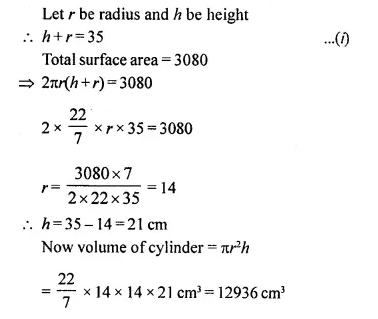Question 22.
The total surface area of a solid cylinder is 616 cm2. If the ratio between its curved surface area and total surface area is 1:2; find the volume of the cylinder.
Solution:
Total surface area of a cylinder = 616 cm2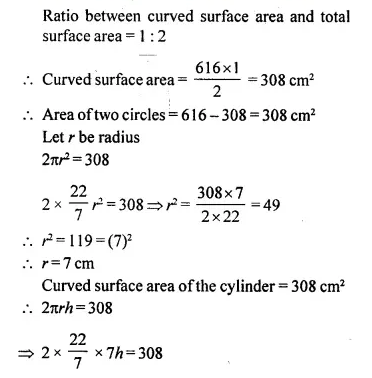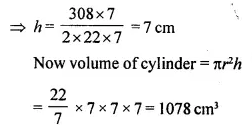Question 23.
A cylindrical vessel of height 24 cm and diameter 40 cm is full of water. Find the exact number of small cylindrical bottles, each of height 10 cm and diameter 8 cm, which can be filled with this water.
Solution:
Height of cylinder (6) = 24 cm
Radius (r)= $$\frac { 40 }{ 2 }$$ = 20 cm
∴ Volume of water filled in it = πr2h
= π x 20 x 20 x 24 cm3
= 9600π cm3
Radius of small cylindrical bottle = $$\frac { 8 }{ 2 }$$ = 4 cm
and height (6) = 10 cm
∴  Volume of one small bottle = πr2h
π x 4 x 4 x 10 cm3 = 160π cm3
∴ Number of small bottles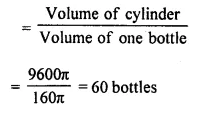Question 24.
Two solid cylinders, one with diameter 60 cm and height 30 cm and the other with radius 30 cm and height 60 cm, are melted and recasted into a third solid cylinder of height 10 cm. Find the diameter of the cylinder formed.
Solution:
Diameter of first cylinder = 60 cm
∴ Radius (R)= $$\frac { 60 }{ 2 }$$ =30 cm
and height (h) = 30 cm
Radius of second cylinder = 30 cm
and height = 60 cm
Volume of first cylinder = πR2h
= π30 x 30 x 30 cm3 = 27000π cm3
and volume of second cylinder = π x 30 x 30 x 60 cm3 = 54000πcm3
Total volume of both cylinders
= 27000π+ 54000π cm3
= 81000π cm3
Volume of third cylinder = 81000π cm3
Height of third cylinder = 10 cm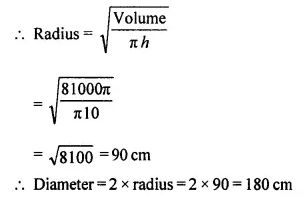Question 25.
The total surface area of a hollow cylinder, which is open from both the sides, is 3575 cm2; area of its base ring is 357.5 cm2 and its height is 14 cm. Find the thickness of the cylinder.
Solution:
Total surface area of an opened hollow cylinder = 3575 cm2
Area of ring of its base = 357.5 cm2
Height = 14 cm
Let R and r be the outer and inner radius of the ring.
∴ π(R2 – r2) = 357.5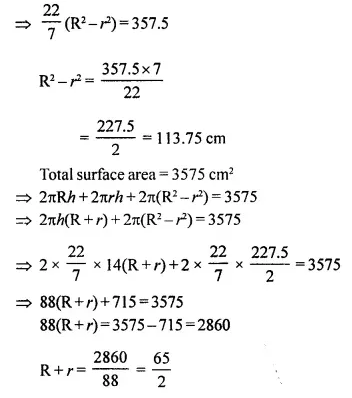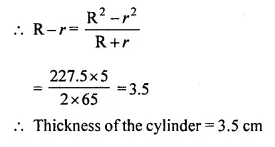Question 26.
The given figure shows a solid formed of a solid cube of side 40 cm and a solid cylinder of radius 20 cm and height 50 cm attached to the cube as shown.
Find the volume and the total surface area of the whole solid [Take π=3.14]Solution:
Side of the cube = 40 cm
Radius of cylinder = 20 cm
Height (h) = 50 cm
Volume of cube = (40)3 = 64000 cm3
and volume of cylinder = πr2h
= 3.14 x 20 x 20 x 50 cm3
=314×200 = 62800 cm3
∴ Total volume = 64000 + 62800 =126800cm3
Total surface area = (6a2 + 2πrh)
= 6 x 40 x 40 + 2 x 3.14 x 20 x 50 =9600+6280
= 15880 cm2

Question 27.
Two right circular solid cylinders have radii in the ratio 3: 5 and heights in the ratio 2:3. Find the ratio between their:
(i) curved surface areas.
(ii) volumes.
Solution:
The ratio is the radii of two right circular solid cylinder = 3:5
and ratio in their heights = 2:3
(i) Let radius of first cylinder = 3x
and height = 2y
∴ Curved surface area = 2πrh
= 2π(3x) (2y) = 12 πxy
and radius of second cylinder = 5x
and height = 3y
∴  Curved surface = 2πrh
= 2π x 5x 3y
= 30πxy
∴ Ratio in their curved surface
= 12πxy : 30πxy
= 2 :5
(ii) Volume of first cylinder = πr2h
= π(3x)2 x 2y = 18πx2y
and volume of second cylinder = π(5x)2 x 3y
= 75πx2y
∴ Ratio = 18πx2y : 75πx2y
= 6:25

Question 28.
A closed cylindrical tank, made of thin iron sheet, has diameter = 8.4 m and height 5.4 m. How much metal sheet, to the nearest m2, is used in making this tank, if $$\frac { 1 }{ 15 }$$ of the sheet actually used was wasted in making the tank ?
Solution:
Diameter of a closed cylindrical tank=8.4 cm
∴ Radius (r) = $$\frac { 8.4 }{ 2 }$$ = 4.2 m
and height (h) = 5.4 m
∴ Total surface area = 2πr(r + h)
= 2 x $$\frac { 22 }{ 7 }$$ x 4.2(4.2+ 5.4) m2
=26.4 x 9.6 m2=253.44 m2
Area of sheet used in wastage
= $$\frac { 1 }{ 15 }$$ of 253.44= 16.896 m2
Total sheet required = 16.896 +253.44 m2
= 270.336 m2
=270.34 m2 (approx)

Hope given Selina Concise Mathematics Class 10 ICSE Solutions Chapter 20 Cylinder, Cone and Sphere Ex 20A are helpful to complete your math homework.

If you have any doubts, please comment below. Learn Insta try to provide online math tutoring for you.